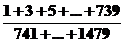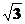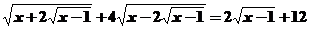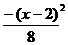WORCESTER POLYTECHNIC INSTITUTE

TWENTIETH ANNUAL INVITATIONAL MATH MEET

OCTOBER 17, 2007

INDIVIDUAL EXAM QUESTION SHEET WITH ANSWERS

1.      What is the 2007th digit in the decimal representation of  1/14?

Ans:   1

2.  Examining the graph of   f(t) = e2tsin(πt)  for  t ≥ 0   where  t  is in seconds, in the first

4 minutes, how many times does the graph cross the  t axis?

Ans:  239

3.  If   ydye = 1   and   y  is not  +/-1,  evaluate  4d –e2 + d2 + 4e -10.

Ans:  -10

4.  It is known that the sum of the first  n  odd integers is  n2.   Determine the simplest form ofAns:  1/3

5.  A triangle has sides   5,  10and  15.   Find the length of the bisector of the second

largest angle.

Ans:  10

6.   A solar reflector is made of 36 triangular sections with sides of 6.2 mm, 6.2 mm and

1.1 mm.  What is the total area of the reflector rounded to the nearest square mm?

Ans:  122 mm2

7.  How many permutations of the digits   0,1,2,..,9   have an even digit in the first place and

1,2,3,4  or  5  in the last place?

Ans:  (2*4 + 3*5)* 8! = 927360

8.  The nth Mersenne Number is given by   Mn = 2n 1    where   n   is a positive integer.  What

is the binary form of   Mn ?

Ans:  111…1  (n times)

9.      For what real values of   x   does the following equation hold?Ans:  x = 26

10.  In the expansion of   (a- 2b)11   what is the coefficient  of   a8b3  ?

Ans:  -1320

11.  A parabola has its focus at  (2,6)  and directrix  the line  y = 10.   What is its equation?

Ans:  y – 8 =